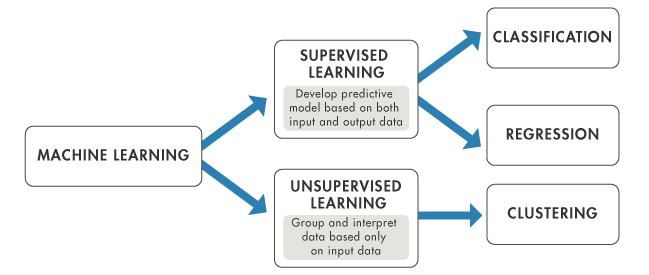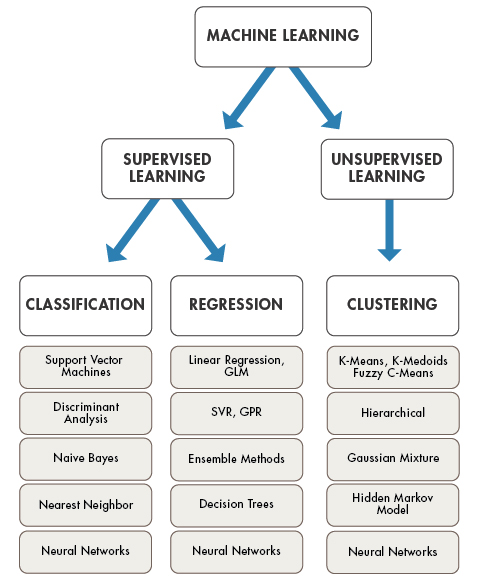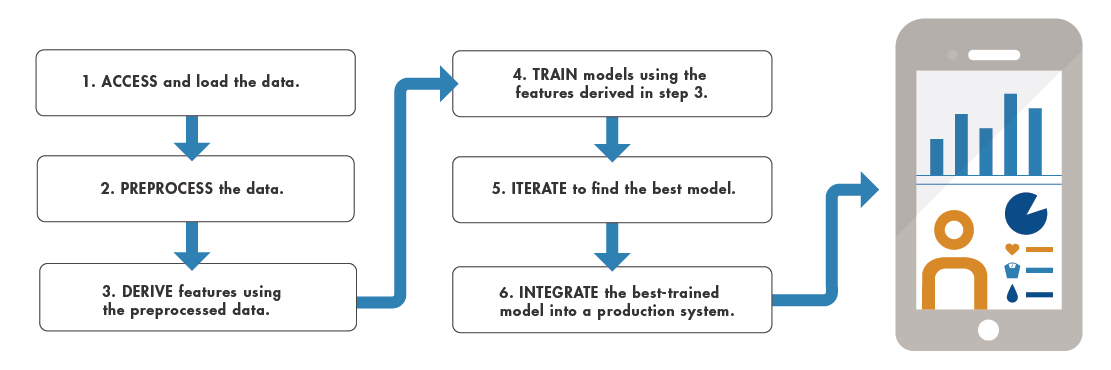## Machine Learning in MATLAB

### What Is Machine Learning?

Machine learning teaches computers to do what comes naturally to humans: learn from experience. Machine learning algorithms use computational methods to “learn” information directly from data without relying on a predetermined equation as a model. The algorithms adaptively improve their performance as the number of samples available for learning increases.

Machine learning uses two types of techniques: supervised learning, which trains a model on known input and output data so that it can predict future outputs, and unsupervised learning, which finds hidden patterns or intrinsic structures in input data.The aim of supervised machine learning is to build a model that makes predictions based on evidence in the presence of uncertainty. A supervised learning algorithm takes a known set of input data and known responses to the data (output) and trains a model to generate reasonable predictions for the response to new data. Supervised learning uses classification and regression techniques to develop predictive models.

• Classification techniques predict categorical responses, for example, whether an email is genuine or spam, or whether a tumor is cancerous or benign. Classification models classify input data into categories. Typical applications include medical imaging, image and speech recognition, and credit scoring.

• Regression techniques predict continuous responses, for example, changes in temperature or fluctuations in power demand. Typical applications include electricity load forecasting and algorithmic trading.

Unsupervised learning finds hidden patterns or intrinsic structures in data. It is used to draw inferences from datasets consisting of input data without labeled responses. Clustering is the most common unsupervised learning technique. It is used for exploratory data analysis to find hidden patterns or groupings in data. Applications for clustering include gene sequence analysis, market research, and object recognition.

### Selecting the Right Algorithm

Choosing the right algorithm can seem overwhelming—there are dozens of supervised and unsupervised machine learning algorithms, and each takes a different approach to learning. There is no best method or one size fits all. Finding the right algorithm is partly based on trial and error—even highly experienced data scientists cannot tell whether an algorithm will work without trying it out. Highly flexible models tend to overfit data by modeling minor variations that could be noise. Simple models are easier to interpret but might have lower accuracy. Therefore, choosing the right algorithm requires trading off one benefit against another, including model speed, accuracy, and complexity. Trial and error is at the core of machine learning—if one approach or algorithm does not work, you try another. MATLAB® provides tools to help you try out a variety of machine learning models and choose the best.To find MATLAB apps and functions to help you solve machine learning tasks, consult the following table. Some machine learning tasks are made easier by using apps, and others use command-line features.

Classification to predict categorical responses

Use the Classification Learner app to automatically train a selection of models and help you choose the best. You can generate MATLAB code to work with scripts.

For more options, you can use the command-line interface.

Statistics and Machine Learning Toolbox™
Regression to predict continuous responses

Use the Regression Learner app to automatically train a selection of models and help you choose the best. You can generate MATLAB code to work with scripts and other function options.

For more options, you can use the command-line interface.

Statistics and Machine Learning Toolbox
ClusteringUse cluster analysis functions.Statistics and Machine Learning ToolboxCluster Analysis
Computational finance tasks such as credit scoringUse tools for modeling credit risk analysis.Financial Toolbox™
Risk Management Toolbox™
Credit Risk (Financial Toolbox)
Deep learning with neural networks for classification and regressionUse pretrained networks and functions to train convolutional neural networks.Deep Learning Toolbox™Deep Learning in MATLAB (Deep Learning Toolbox)
Facial recognition, motion detection, and object detectionUse deep learning tools for image processing and computer vision.Deep Learning Toolbox
Computer Vision Toolbox™
Deep Learning, Semantic Segmentation, and Detection (Computer Vision Toolbox)

The following systematic machine learning workflow can help you tackle machine learning challenges. You can complete the entire workflow in MATLAB.To integrate the best trained model into a production system, you can deploy Statistics and Machine Learning Toolbox machine learning models using MATLAB Compiler™. For many models, you can generate C-code for prediction using MATLAB Coder™.

### Train Classification Models in Classification Learner App

Use the Classification Learner app to train models to classify data using supervised machine learning. The app lets you explore supervised machine learning interactively using various classifiers.

• Automatically train a selection of models to help you choose the best model. Model types include decision trees, discriminant analysis, support vector machines, logistic regression, nearest neighbors, naive Bayes, and ensemble classification.

• Explore your data, specify validation schemes, select features, and visualize results. By default, the app protects against overfitting by applying cross-validation. Alternatively, you can select holdout validation. Validation results help you choose the best model for your data. Plots and performance measures reflect the validated model results.

• Export models to the workspace to make predictions with new data. The app always trains a model on full data in addition to a model with the specified validation scheme, and the full model is the model you export.

• Generate MATLAB code from the app to create scripts, train with new data, work with huge data sets, or modify the code for further analysis.For more options, you can use the command-line interface. See Classification.

### Train Regression Models in Regression Learner App

Use the Regression Learner app to train models to predict continuous data using supervised machine learning. The app lets you explore supervised machine learning interactively using various regression models.

• Automatically train a selection of models to help you choose the best model. Model types include linear regression models, regression trees, Gaussian process regression models, support vector machines, and ensembles of regression trees.

• Explore your data, select features, and visualize results. Similar to Classification Learner, the Regression Learner applies cross-validation by default. The results and visualizations reflect the validated model. Use the results to choose the best model for your data.

• Export models to the workspace to make predictions with new data. The app always trains a model on full data in addition to a model with the specified validation scheme, and the full model is the model you export.

• Generate MATLAB code from the app to create scripts, train with new data, work with huge data sets, or modify the code for further analysis.For more options, you can use the command-line interface. See Regression.

### Train Neural Networks for Deep Learning

Deep Learning Toolbox enables you to perform deep learning with convolutional neural networks for classification, regression, feature extraction, and transfer learning. The toolbox provides simple MATLAB commands for creating and interconnecting the layers of a deep neural network. Examples and pretrained networks make it easy to use MATLAB for deep learning, even without extensive knowledge of advanced computer vision algorithms or neural networks.